# 978-0073382395 Chapter 9 Questions and Problems 16-28

Document Type
Homework Help
Book Title
Fundamentals of Corporate Finance Standard Edition 9th Edition
Authors
Stephen Ross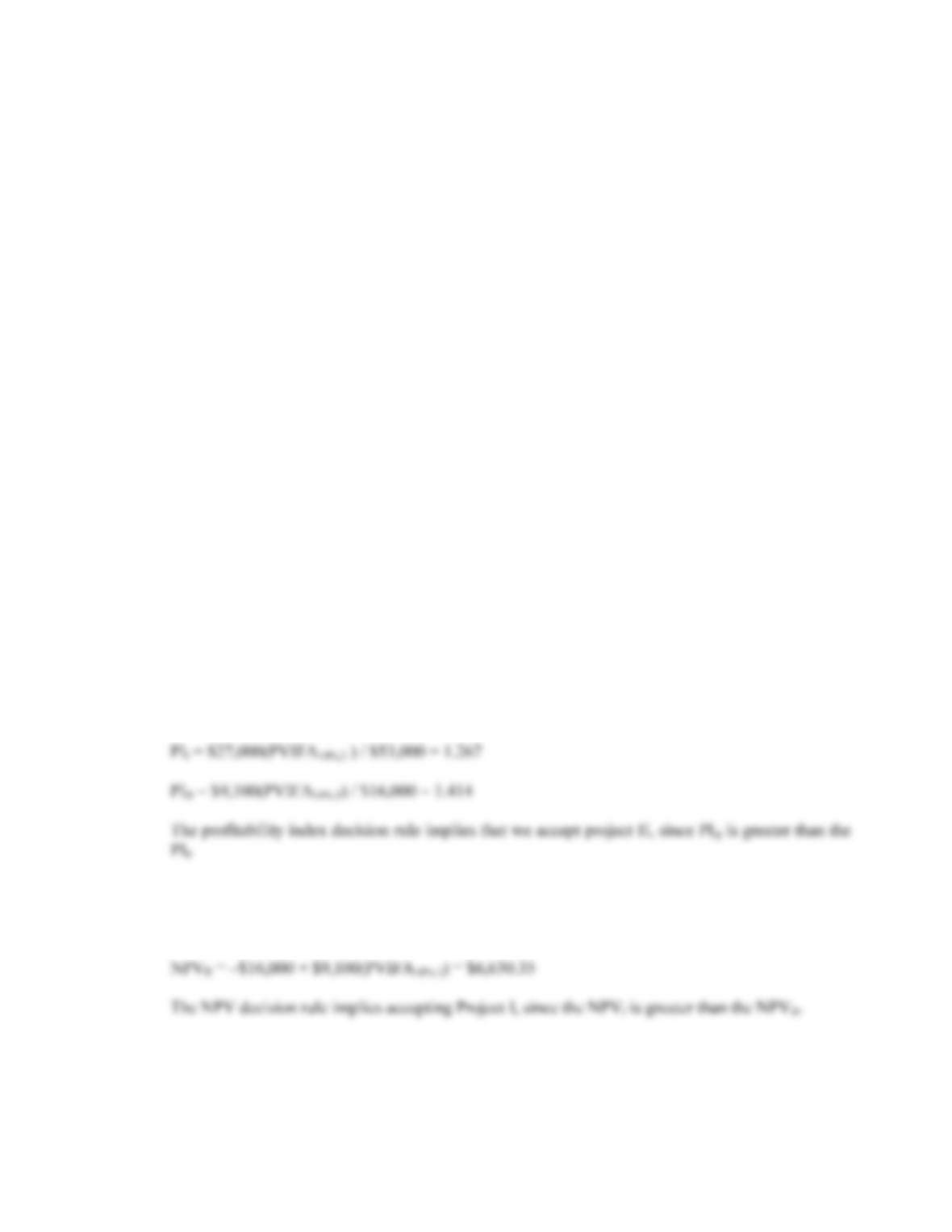CHAPTER 9 B-163
b. The equation for the IRR of the project is:
0 = –\$45,000,000 + \$78,000,000/(1+IRR) – \$14,000,000/(1+IRR)2
From Descartes rule of signs, we know there are potentially two IRRs since the cash flows change
signs twice. From trial and error, the two IRRs are:
IRR = 53.00%, –79.67%
When there are multiple IRRs, the IRR decision rule is ambiguous. Both IRRs are correct, that is,
both interest rates make the NPV of the project equal to zero. If we are evaluating whether or not
to accept this project, we would not want to use the IRR to make our decision.
15. The profitability index is defined as the PV of the cash inflows divided by the PV of the cash outflows.
The equation for the profitability index at a required return of 10 percent is:
PI = [\$7,300/1.1 + \$6,900/1.12 + \$5,700/1.13] / \$14,000 = 1.187
The equation for the profitability index at a required return of 15 percent is:
PI = [\$7,300/1.15 + \$6,900/1.152 + \$5,700/1.153] / \$14,000 = 1.094
The equation for the profitability index at a required return of 22 percent is:
PI = [\$7,300/1.22 + \$6,900/1.222 + \$5,700/1.223] / \$14,000 = 0.983
We would accept the project if the required return were 10 percent or 15 percent since the PI is greater
than one. We would reject the project if the required return were 22 percent since the PI is less than one.
16. a. The profitability index is the PV of the future cash flows divided by the initial investment. The cash
flows for both projects are an annuity, so:
b. The NPV of each project is:
NPVI = –\$53,000 + \$27,000(PVIFA10%,3) = \$14,145.00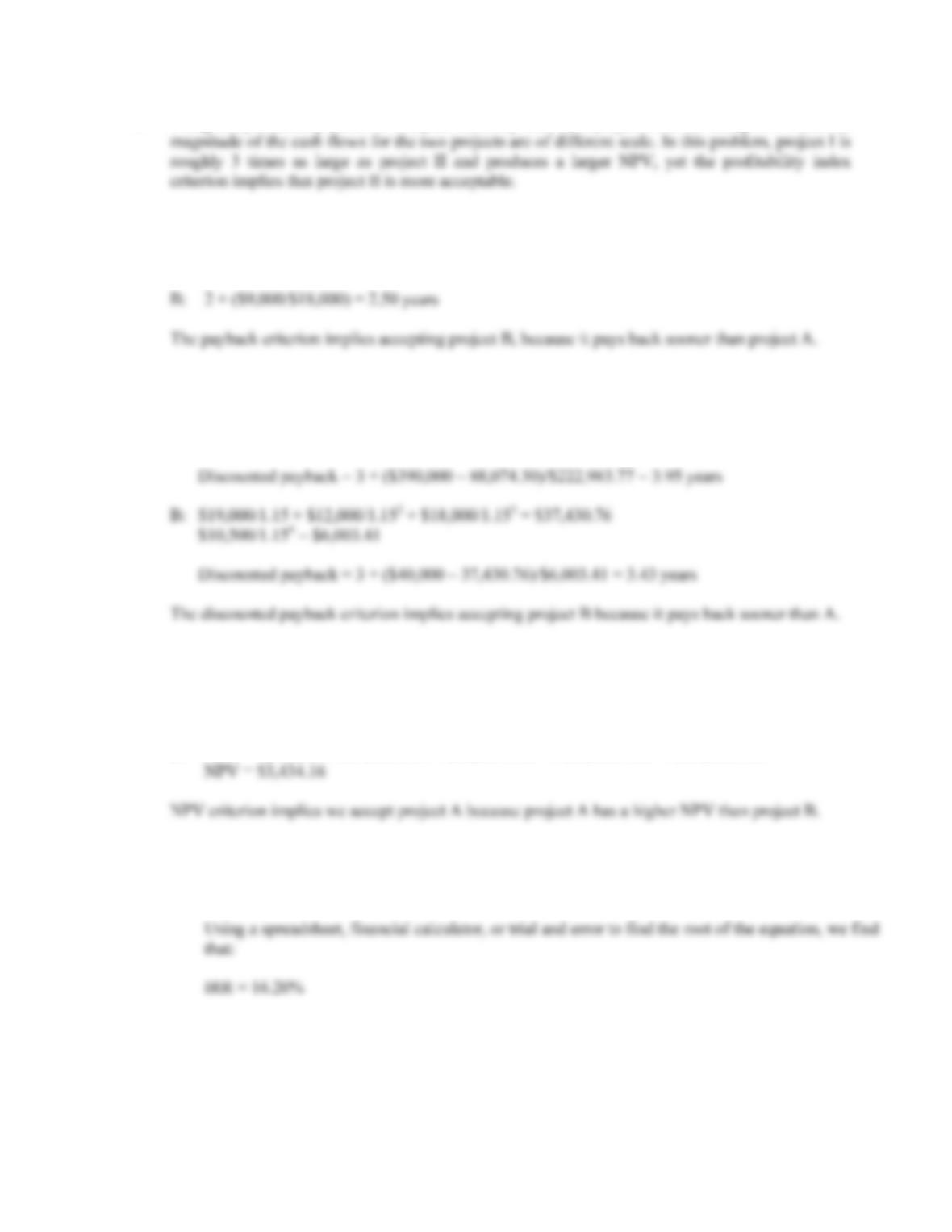B-164 SOLUTIONS
c. Using the profitability index to compare mutually exclusive projects can be ambiguous when the
17. a. The payback period for each project is:
A: 3 + (\$180,000/\$390,000) = 3.46 years
b. The discounted payback for each project is:
A: \$20,000/1.15 + \$50,000/1.152 + \$50,000/1.153 = \$88,074.30
\$390,000/1.154 = \$222,983.77
c. The NPV for each project is:
A: NPV = –\$300,000 + \$20,000/1.15 + \$50,000/1.152 + \$50,000/1.153 + \$390,000/1.154
NPV = \$11,058.07
B: NPV = –\$40,000 + \$19,000/1.15 + \$12,000/1.152 + \$18,000/1.153 + \$10,500/1.154
d. The IRR for each project is:
A: \$300,000 = \$20,000/(1+IRR) + \$50,000/(1+IRR)2 + \$50,000/(1+IRR)3 + \$390,000/(1+IRR)4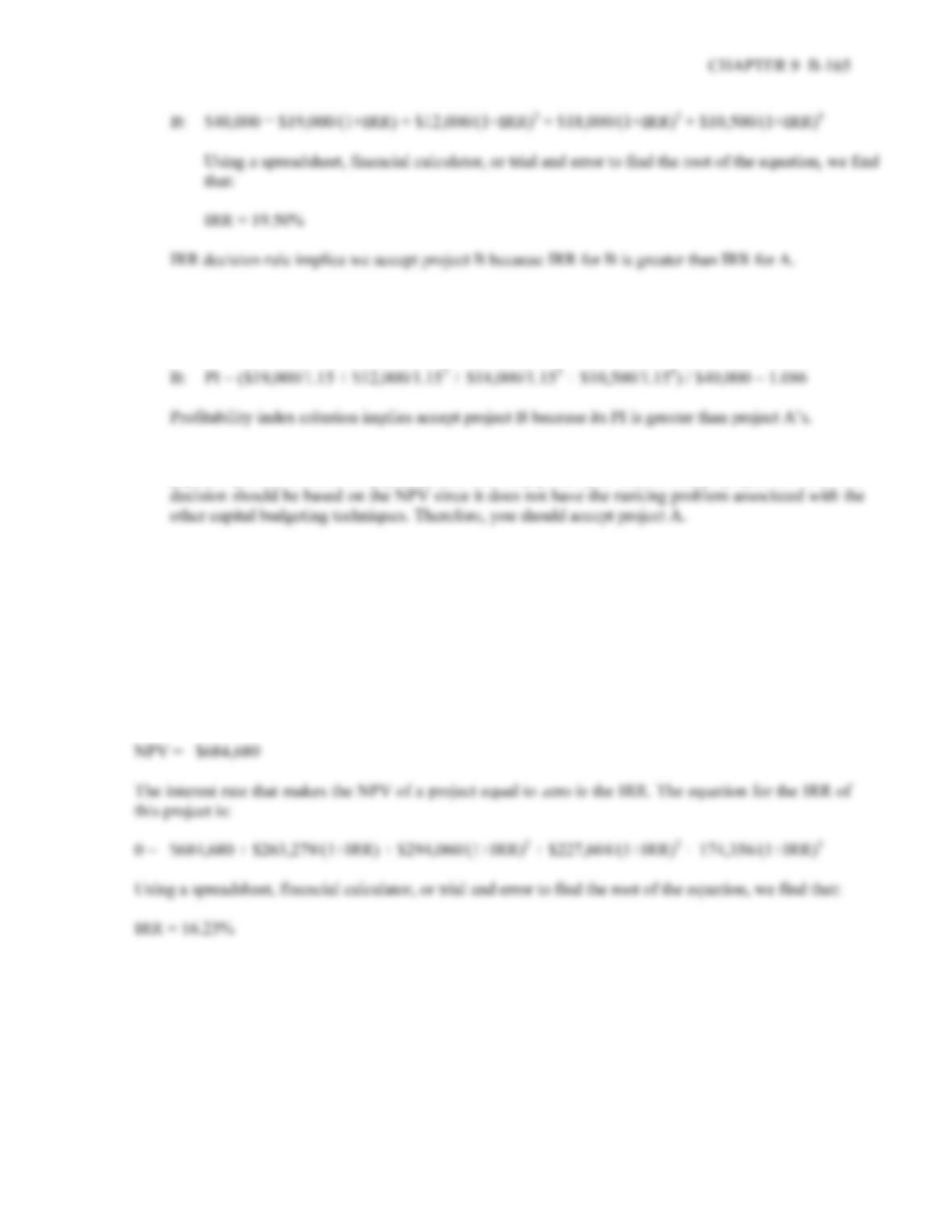e. The profitability index for each project is:
A: PI = (\$20,000/1.15 + \$50,000/1.152 + \$50,000/1.153 + \$390,000/1.154) / \$300,000 = 1.037
f. In this instance, the NPV criteria implies that you should accept project A, while profitability index,
payback period, discounted payback, and IRR imply that you should accept project B. The final
18. At a zero discount rate (and only at a zero discount rate), the cash flows can be added together across
time. So, the NPV of the project at a zero percent required return is:
NPV = –\$684,680 + 263,279 + 294,060 + 227,604 + 174,356 = \$274,619
If the required return is infinite, future cash flows have no value. Even if the cash flow in one year is \$1
trillion, at an infinite rate of interest, the value of this cash flow today is zero. So, if the future cash
flows have no value today, the NPV of the project is simply the cash flow today, so at an infinite
interest rate: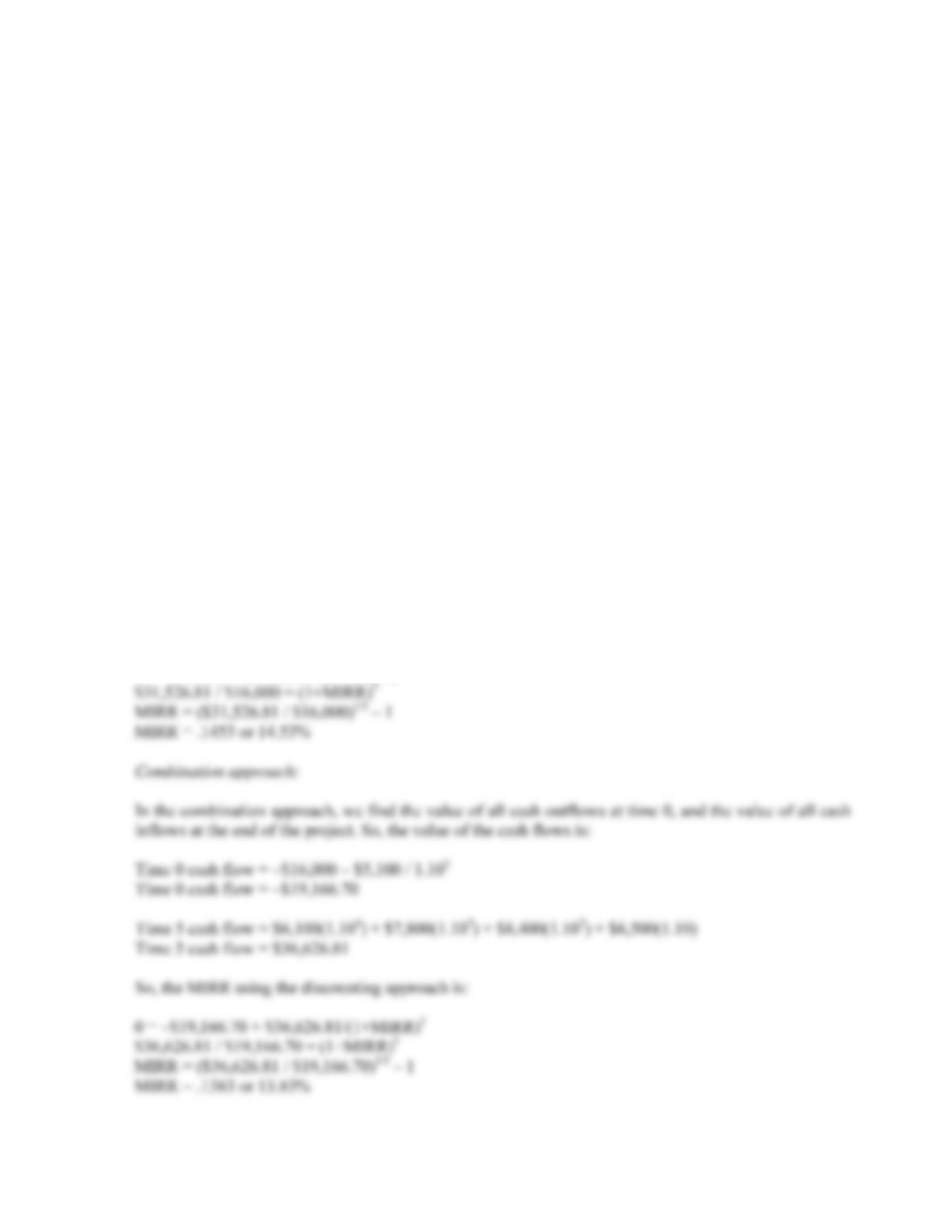B-166 SOLUTIONS
19. The MIRR for the project with all three approaches is:
Discounting approach:
In the discounting approach, we find the value of all cash outflows to time 0, while any cash inflows
remain at the time at which they occur. So, the discounting the cash outflows to time 0, we find:
Time 0 cash flow = –\$16,000 – \$5,100 / 1.105
Time 0 cash flow = –\$19,166.70
So, the MIRR using the discounting approach is:
0 = –\$19,166.70 + \$6,100/(1+MIRR) + \$7,800/(1+MIRR)2 + \$8,400/(1+MIRR)3 + 6,500/(1+MIRR)4
Using a spreadsheet, financial calculator, or trial and error to find the root of the equation, we find that:
MIRR = 18.18%
Reinvestment approach:
In the reinvestment approach, we find the future value of all cash except the initial cash flow at the end
of the project. So, reinvesting the cash flows to time 5, we find:
Time 5 cash flow = \$6,100(1.104) + \$7,800(1.103) + \$8,400(1.102) + \$6,500(1.10) – \$5,100
Time 5 cash flow = \$31,526.81
So, the MIRR using the discounting approach is:
0 = –\$16,000 + \$31,526.81/(1+MIRR)5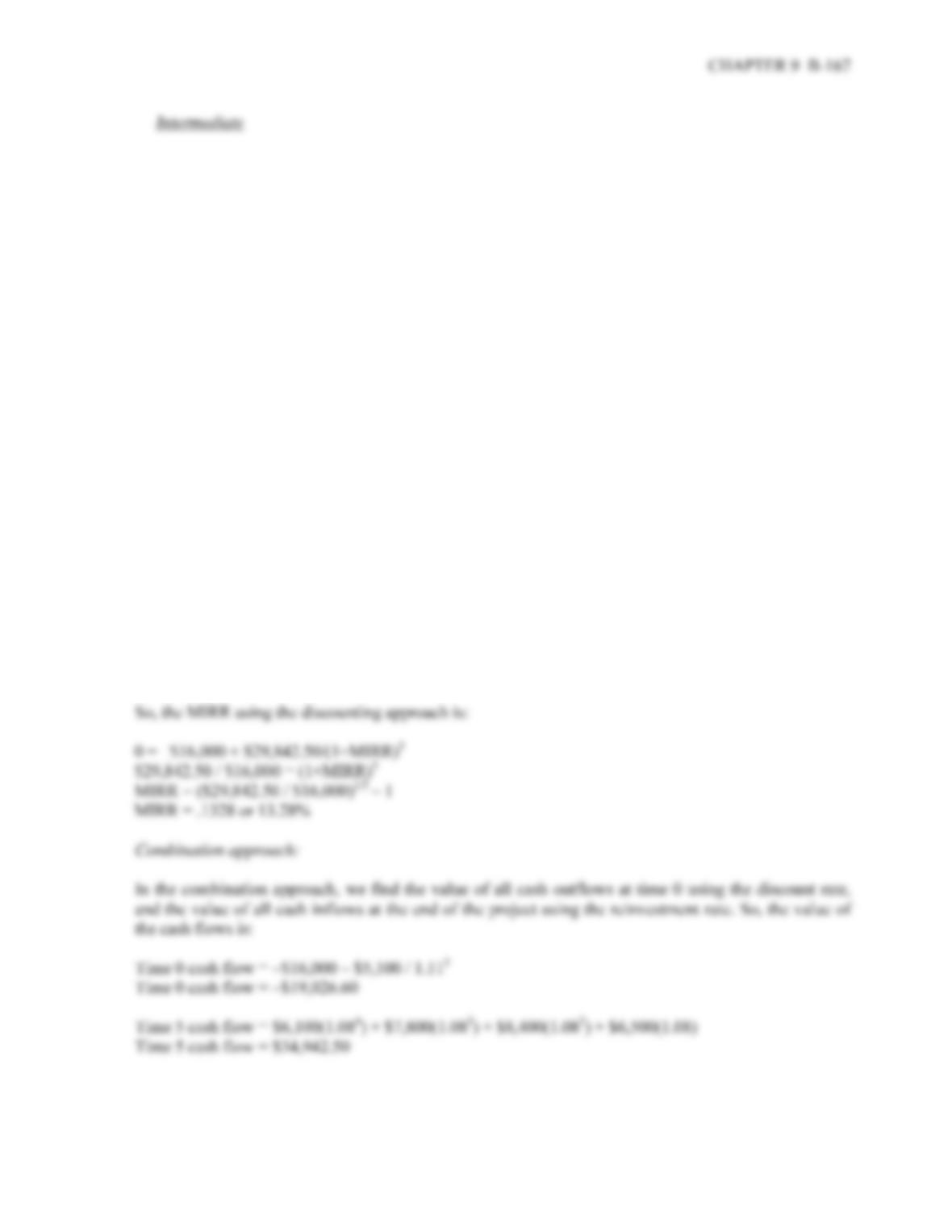20. With different discounting and reinvestment rates, we need to make sure to use the appropriate interest
rate. The MIRR for the project with all three approaches is:
Discounting approach:
In the discounting approach, we find the value of all cash outflows to time 0 at the discount rate, while
any cash inflows remain at the time at which they occur. So, the discounting the cash outflows to time 0,
we find:
Time 0 cash flow = –\$16,000 – \$5,100 / 1.115
Time 0 cash flow = –\$19,026.60
So, the MIRR using the discounting approach is:
0 = –\$19,026.60 + \$6,100/(1+MIRR) + \$7,800/(1+MIRR)2 + \$8,400/(1+MIRR)3 + 6,500/(1+MIRR)4
Using a spreadsheet, financial calculator, or trial and error to find the root of the equation, we find that:
MIRR = 18.55%
Reinvestment approach:
In the reinvestment approach, we find the future value of all cash except the initial cash flow at the end
of the project using the reinvestment rate. So, the reinvesting the cash flows to time 5, we find:
Time 5 cash flow = \$6,100(1.084) + \$7,800(1.083) + \$8,400(1.082) + \$6,500(1.08) – \$5,100
Time 5 cash flow = \$29,842.50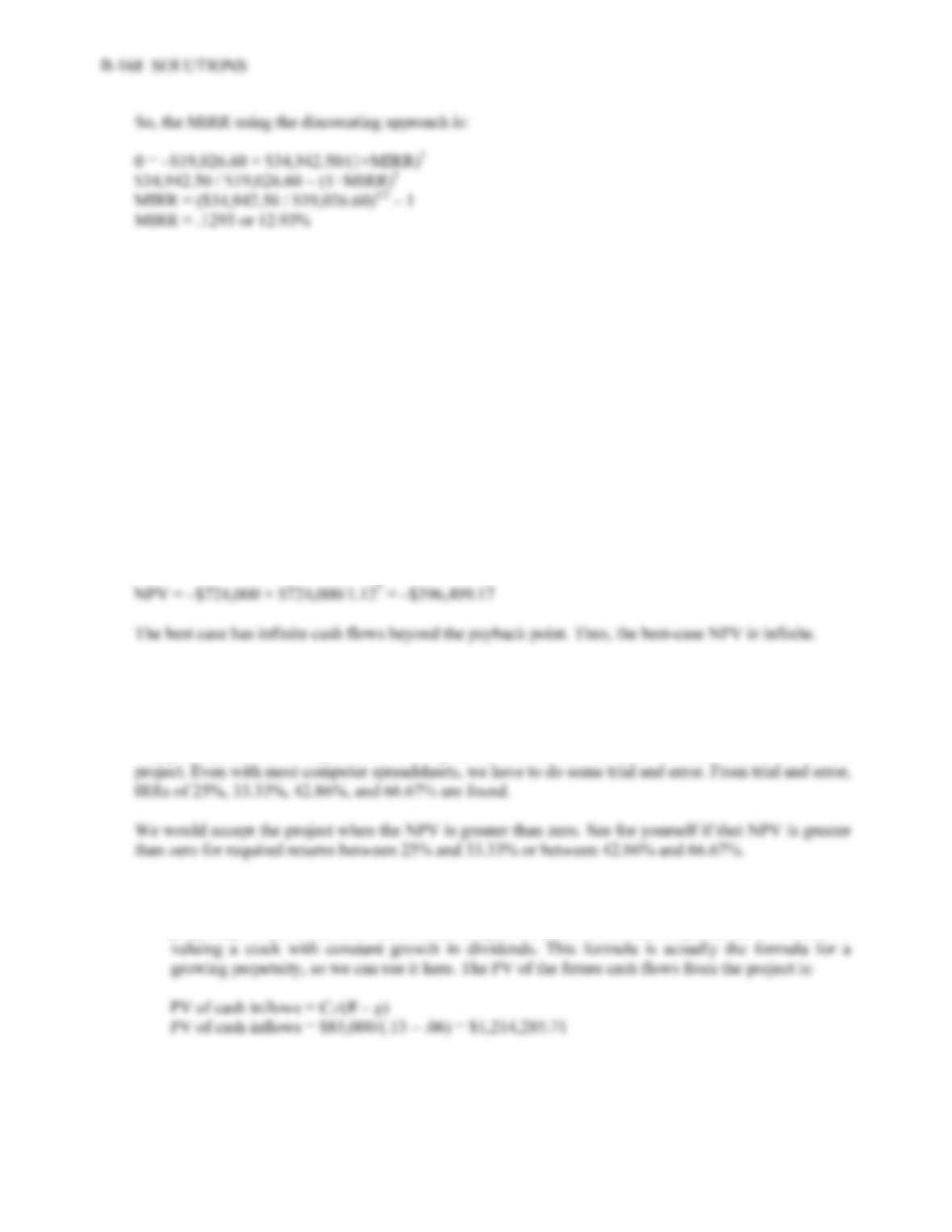21. Since the NPV index has the cost subtracted in the numerator, NPV index = PI – 1.
22. a. To have a payback equal to the project’s life, given C is a constant cash flow for N years:
C = I/N
b. To have a positive NPV, I < C (PVIFAR%, N). Thus, C > I / (PVIFAR%, N).
c. Benefits = C (PVIFAR%, N) = 2 × costs = 2I
C = 2I / (PVIFAR%, N)
Challenge
23. Given the seven year payback, the worst case is that the payback occurs at the end of the seventh year.
Thus, the worst-case:
24. The equation for the IRR of the project is:
0 = –\$1,512 + \$8,586/(1 + IRR) – \$18,210/(1 + IRR)2 + \$17,100/(1 + IRR)3 – \$6,000/(1 + IRR)4
Using Descartes rule of signs, from looking at the cash flows we know there are four IRRs for this
25. a. Here the cash inflows of the project go on forever, which is a perpetuity. Unlike ordinary
perpetuity cash flows, the cash flows here grow at a constant rate forever, which is a growing
perpetuity. If you remember back to the chapter on stock valuation, we presented a formula for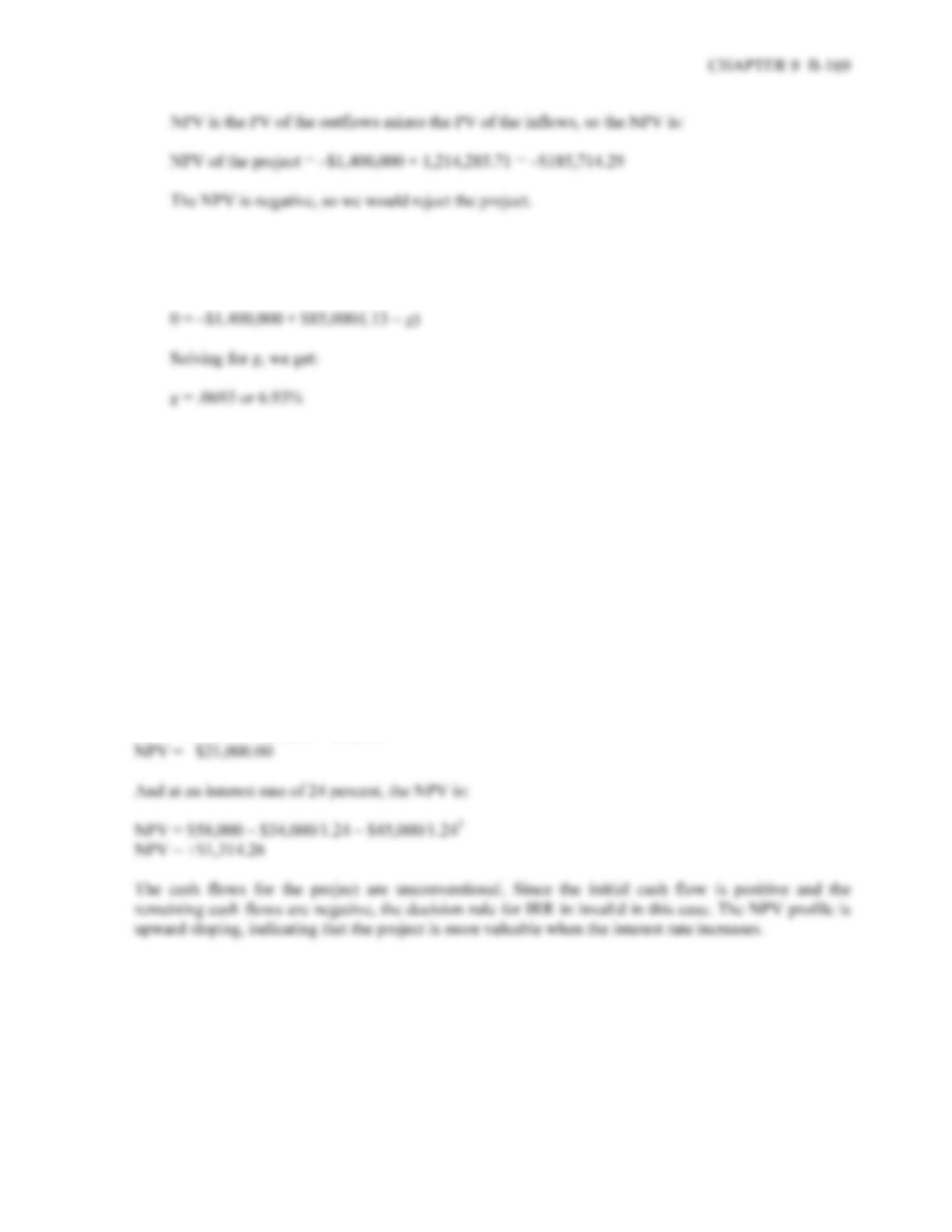b. Here we want to know the minimum growth rate in cash flows necessary to accept the project. The
minimum growth rate is the growth rate at which we would have a zero NPV. The equation for a
zero NPV, using the equation for the PV of a growing perpetuity is:
26. The IRR of the project is:
\$58,000 = \$34,000/(1+IRR) + \$45,000/(1+IRR)2
Using a spreadsheet, financial calculator, or trial and error to find the root of the equation, we find that:
IRR = 22.14%
At an interest rate of 12 percent, the NPV is:
NPV = \$58,000 – \$34,000/1.12 – \$45,000/1.122
NPV = –\$8,230.87
At an interest rate of zero percent, we can add cash flows, so the NPV is:
NPV = \$58,000 – \$34,000 – \$45,000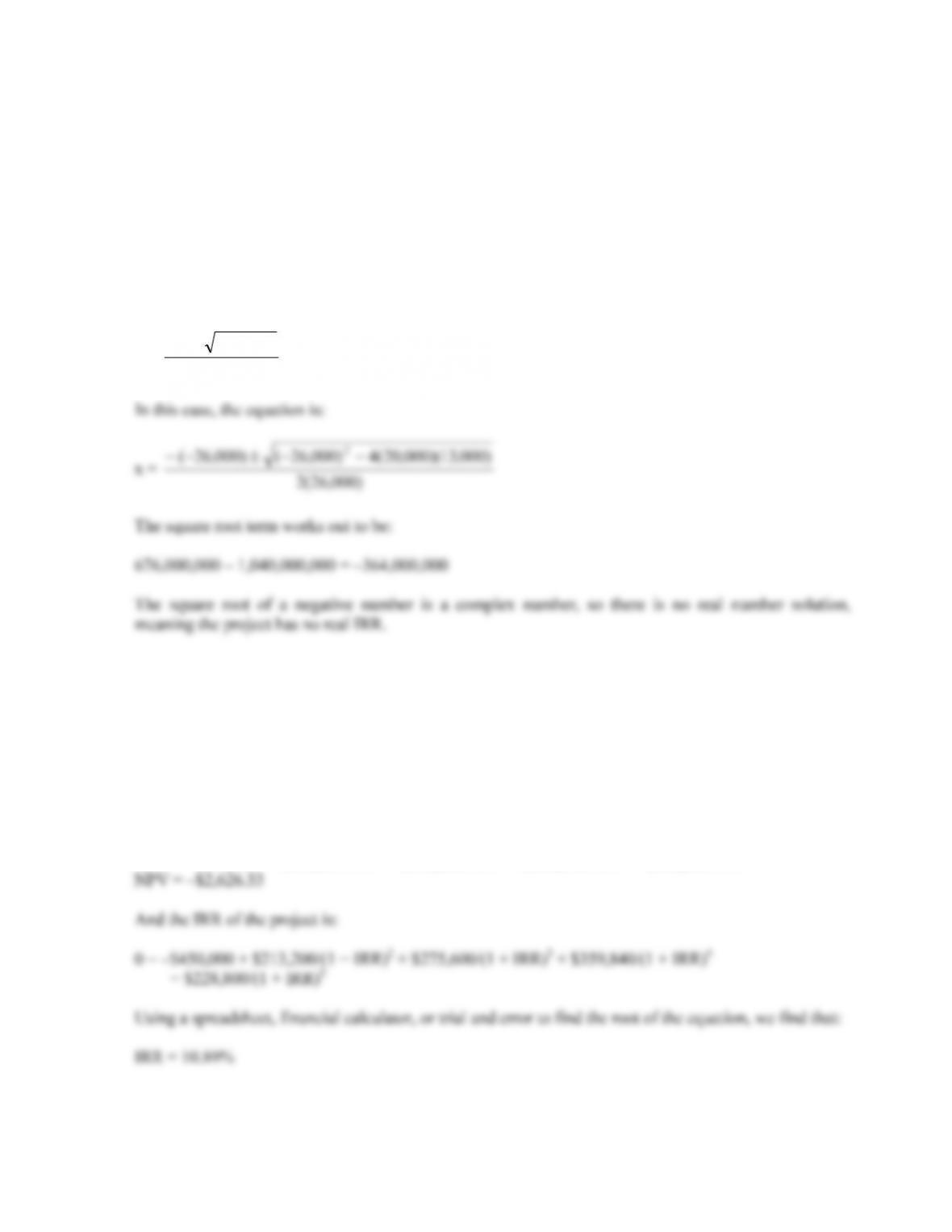B-170 SOLUTIONS
27. The IRR is the interest rate that makes the NPV of the project equal to zero. So, the IRR of the project
is:
0 = \$20,000 – \$26,000 / (1 + IRR) + \$13,000 / (1 + IRR)2
Even though it appears there are two IRRs, a spreadsheet, financial calculator, or trial and error will not
give an answer. The reason is that there is no real IRR for this set of cash flows. If you examine the IRR
equation, what we are really doing is solving for the roots of the equation. Going back to high school
algebra, in this problem we are solving a quadratic equation. In case you don’t remember, the quadratic
equation is:
x = a
acbb
2
4
2
28. First, we need to find the future value of the cash flows for the one year in which they are blocked by
the government. So, reinvesting each cash inflow for one year, we find:
Year 2 cash flow = \$205,000(1.04) = \$213,200
Year 3 cash flow = \$265,000(1.04) = \$275,600
Year 4 cash flow = \$346,000(1.04) = \$359,840
Year 5 cash flow = \$220,000(1.04) = \$228,800
So, the NPV of the project is:
NPV = –\$450,000 + \$213,200/1.112 + \$275,600/1.113 + \$359,840/1.114 + \$228,800/1.115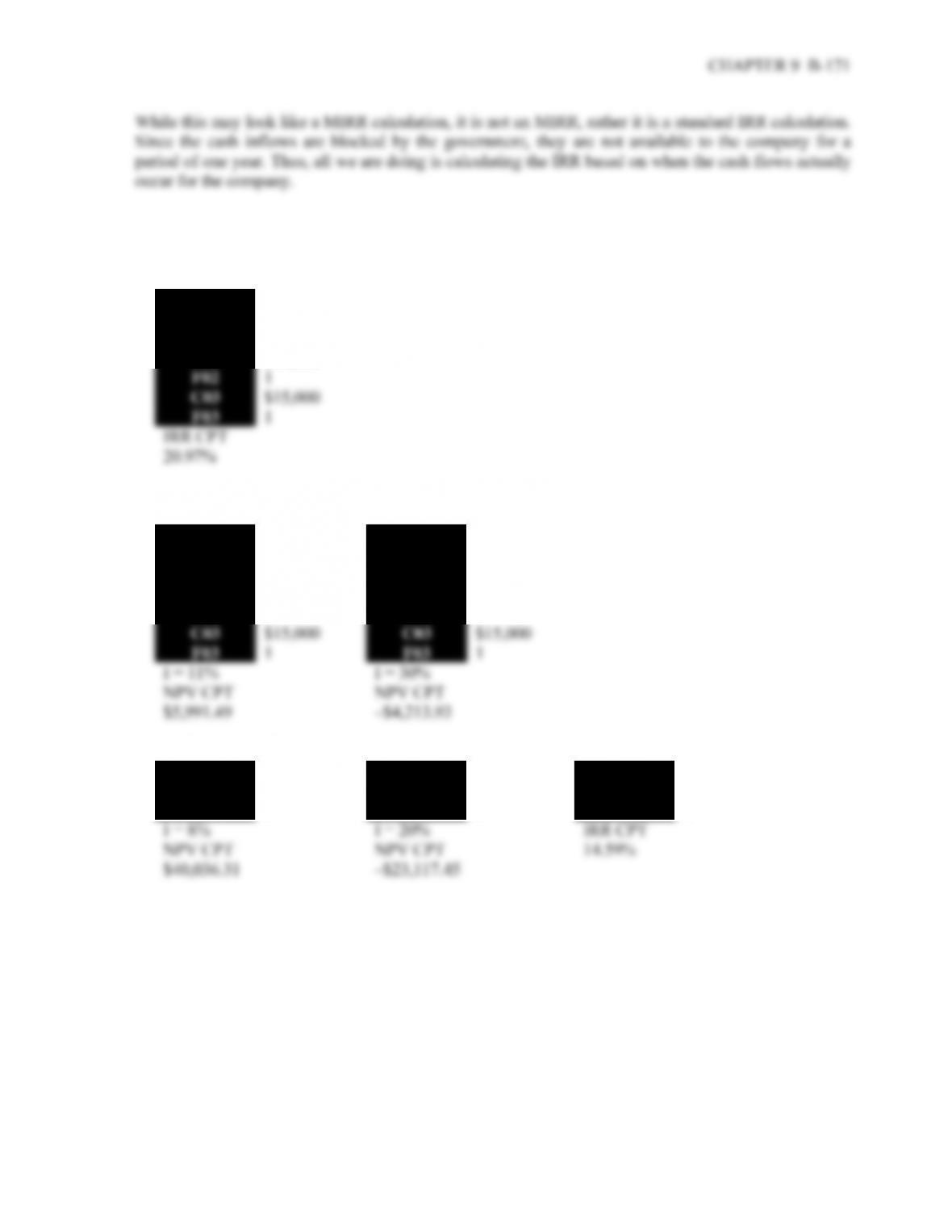Calculator Solutions
7.
CFo –\$34,000
C01 \$16,000
F01 1
C02 \$18,000
8.
CFo –\$34,000 CFo –\$34,000
C01 \$16,000 C01 \$16,000
F01 1 F01 1
C02 \$18,000 C02 \$18,000
F02 1 F02 1
9.
CFo –\$138,000 CFo –\$138,000 CFo –\$138,000
C01 \$28,500 C01 \$28,500 C01 \$28,500
F01 9 F01 9 F01 9

## Trusted by Thousands ofStudents

Here are what students say about us.